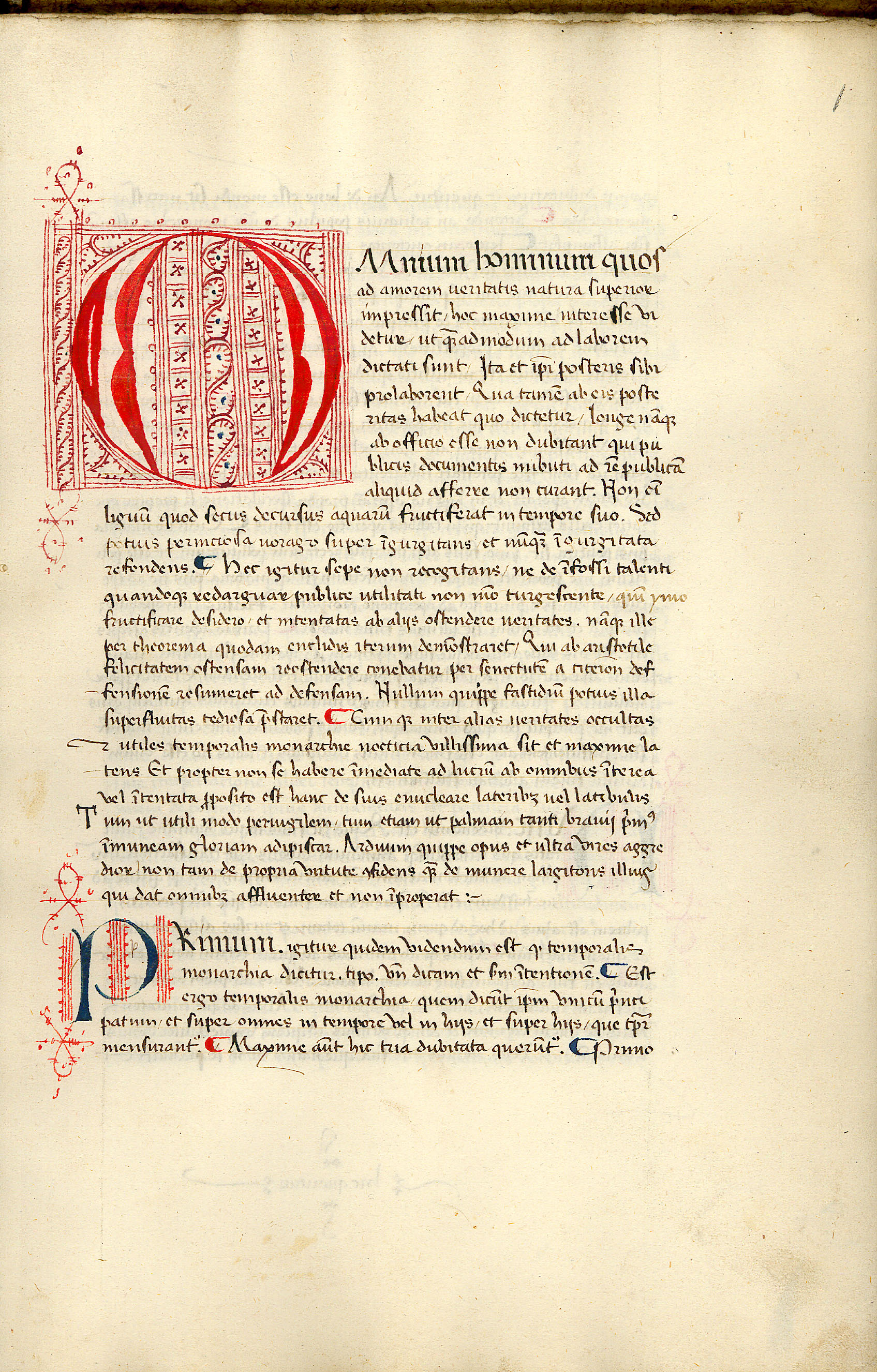Folio 1r       ParagraphA 1r : Book I i 1 - ii 3

#### 1.1.1.

[I][i] OMnium hominum quos
impressit hoc maxime interesse vi
dictati sunt Ita et ipsi posteris sibi
prolaborent Qua tamen ab eis poste
ritas habeat quo dictetur  longe namque
ab officio esse non dubitant qui pu
blicis documentis imbuti ad rem publicam
aliquid afferre non curant Non enim
lignum quod secus decursus aquarum fructiferat in tempore suo Sed
potius perniciosa vorago super ingurgitans et nunquam ingurgitata
refondens  Hec igitur sepe non recogitans ne de infossi talenti
quandoque redarguar publice utilitati non modo turgescente quin ymo
fructificare desidero et intentatas ab aliis ostendere veritates  namque ille
per theorema quodam enclidis iterum demonstraret Qui ab aristotile
felicitatem ostensam reostendere conebatur per senectutem a cicerone def
fensionem resumeret ad defensam Nullum quippe fastidium potius illa
superfluitas tediosa prestaret  Cumque inter alias veritates occultas
et utiles temporalis monarchie nocticia villissima sit et maxime la
tens Et propter non se habere inmediate ad lucrum ab omnibus interea
vel intentata proposito est hanc de suis enucleare lateribus vel latibulis
Tum ut utili modo pervigilem tum etiam ut palmam tanti bravii primus
inmuneam gloriam adipiscar  Arduum quippe opus et ultra vires aggre
dior non tam de propria virtute confidens quam de munere largitoris illius
qui dat omnibus affluenter et non improperat

#### 1.1.2.

[ii] PRimum igitur quidem videndum est quod temporalis
monarchia dicitur tipo unde dicam et secundum intentionem  Est
ergo temporalis monarchia quem dicunt ipsum unicum princi
patum et super omnes in tempore vel in hiis et super hiis que tempore
mensurantur  Maxime autem hic tria dubitata queruntur Primo
Apparatus for Book I, chapter i, paragraph 1
Omnium Ed A B C D E F G H K L M N P Ph Q R S T U V Y Z
hominum Ed A B C D E F G H K L M N P Ph Q R S T U V Y
om.Z
quos ad amorem veritatis Ed A B C E F G H K L N P Q R T U V Y Z
in quos amorem veritatis D
in quos Amorem virtutis M
quos amorem veritatis S
natura Ed A B C D E F G H K L M N P Ph Q R S T U V Y Z
superior Ed A B C D E F G H K L M P Ph Q R S T U V Y Z
om.N
impressit Ed A B C D E F G H K L M N P Ph Q R S T U V Y Z
hoc Ed A B C D E F G H K L M N P Ph Q R S T U V Y Z
maxime Ed A B C D E F G H K L M N P Ph Q R S T U V Y Z
interesse Ed A B C D E F G H K L M N P Ph Q R S T U V Y Z
videtur Ed A B C D E F G H K L M N P Ph Q R S T U V Y Z
ut Ed A C D E F G H K M N Ph R T U V Y Z
Et S
om.B L P Q
quemadmodum Ed A B C D E F G H K L M N P Ph Q R S T U V Y Z
de Ed B C D E F G H K L M N P Ph Q R S T U V Y Z
labore Ed B C D E F G H K L M N P Ph Q R S T U V Y Z
laborem A
antiquorum Ed B C D E F G H K L M N P Ph Q R T U V Y Z
autem eorum S
om.A
ditati Ed B D E F G H K L M N P Ph Q R S T U V Y Z
dictati A
dati C
sunt Ed A B C D E F G H K L M N Ph Q R S T U V Y Z
sumus P
ita Ed A B C D E F G H K L M N P Ph Q R S T U V Y Z
et Ed A C D E F G H K M N P Ph R S T U V Y Z
om.B L Q
ipsi Ed A B C D F G H K L M N P Ph Q S T U V Y Z
ipsis E R
posteris prolaborent Ed B C D F G H L N Ph Q U V Y Z
posteris sibi prolaborent A
posteũs prolaborent E R
pro posteris laborent K T
\pro/ posteri\s/prolaborent M
posteri prolaborent P
posteris perlaborent S
quatenus Ed D E G H K L N P Q R S T U V Y Z
Qua tamen A
quare B
quod C
quibus F
quantum M
quarum Ph
ab Ed A B C D E F G H K L M N P Ph Q R S T U V Y Z
eis Ed A B C D E F G H K L M N P Ph Q R S T U V Y Z
posteritas Ed A B C D E F G H K L M N P Ph Q R S T U V Y Z
habeat Ed A B C D E F G H K L M N P Ph Q R T U V Y Z
abeat S
quo Ed A B C D E F G H K L M P Ph Q R S T U V Y Z
quod N
ditetur Ed B C D E F G H K L M P Q R T U V Y Z
dictetur A
dicetur N Ph
ditentur S